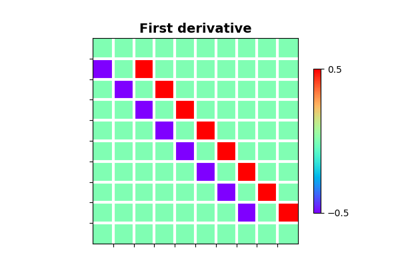# pylops.SecondDirectionalDerivative¶

pylops.SecondDirectionalDerivative(dims, v, sampling=1, edge=False, dtype='float64')[source]

Second Directional derivative.

Apply a second directional derivative operator to a multi-dimensional array along either a single common direction or different directions for each point of the array.

Note

At least 2 dimensions are required, consider using pylops.SecondDerivative for 1d arrays.

Parameters: dims : tuple Number of samples for each dimension. v : np.ndarray, optional Single direction (array of size $$n_\text{dims}$$) or group of directions (array of size $$[n_\text{dims} \times n_{d_0} \times ... \times n_{d_{n_\text{dims}}}]$$) sampling : tuple, optional Sampling steps for each direction. edge : bool, optional Use reduced order derivative at edges (True) or ignore them (False). dtype : str, optional Type of elements in input array. ddop : pylops.LinearOperator Second directional derivative linear operator

Notes

The SecondDirectionalDerivative applies a second-order derivative to a multi-dimensional array along the direction defined by the unitary vector $$\mathbf{v}$$:

$d^2f_\mathbf{v} = - D_\mathbf{v}^T [D_\mathbf{v} f]$

where $$D_\mathbf{v}$$ is the first-order directional derivative implemented by pylops.SecondDirectionalDerivative.

This operator is sometimes also referred to as directional Laplacian in the literature.

## Examples using pylops.SecondDirectionalDerivative¶Derivatives